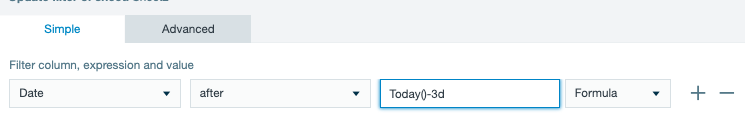•Hello Kang.

What exactly issue do you observe while trying to get "current_date minus 3 days" at a regular Worksheet?

It is much easier to compute this at a regular Worksheet via the formula IF(AFTER(#DateValue;TODAY()-3d);1;0). It returns 1 if a date value is greater than current_date minus 3 days and 0 if otherwise.

Alternatively, you could apply a filter to the desired date column with TODAY()-3d as criteria.•Thanks Konsta. I was actually asking about how to do it using SQL.

The regular worksheets work fine. I just find it easier working with SQL if these functions are available.

•Hello Kang.
Thank you for the additional details.

In a SQL Worksheet, you could use CURRENT_DATE and INTERVAL functions to achieve the desired goal.

`SELECT Sheet.Column FROM Sheet WHERE CAST(Sheet.Column AS DATE ) > CURRENT_DATE - INTERVAL '3' DAY `

Please let me know if this helps.

•Thanks Kosta!  The Interval calc worked.

Another question, how can i subtract one date field from another to get the number of days difference?

When I tried:

Select sheet.ColumnDate - CURRENT_DATE

I get:

Cannot apply '-' to arguments of type '<DATE> - <DATE>'. Supported form(s): '<NUMERIC> - <NUMERIC>' '<DATETIME_INTERVAL> - <DATETIME_INTERVAL>' '<DATETIME> - <DATETIME_INTERVAL>'

•Hello Kang.

To subtract one date field from another you could leverage TIMESTAMPDIFF function. It returns the number of whole date intervals between two date arguments.

Example1. Get the time difference in days between two date values

`SELECT TIMESTAMPDIFF(DAY, Sheet.ColumnDate1, Sheet.ColumnDate2) FROM Sheet`

Example2. Get the time difference in days between a date column and the current date.

`SELECT TIMESTAMPDIFF(DAY, Sheet.ColumnDate, CURRENT_DATE) FROM Sheet`

Please let me know if this helps.

•This did it!  Thanks a lot Kosta!

I had trouble finding examples in the online documentation.  Not sure if i was looking in the right place, but would like to suggest adding date function examples in the SQL Worksheet functions section...especially if not all date worksheet functions are available.  There appears to be different formats for this function..in the documentation, it uses semicolon as the separator instead of comma.

Appreciate the assist!

•Hello Kang.
I'm glad to hear that you were able to proceed further with your project.

Thank you very much for the feedback. I'll check what we could do to make functions usage in a SQL Worksheet more straightforward.

From my understanding, functions available at a SQL Worksheet mainly retain their SQL syntax. The documentation page I've mentioned describes the traditional function.